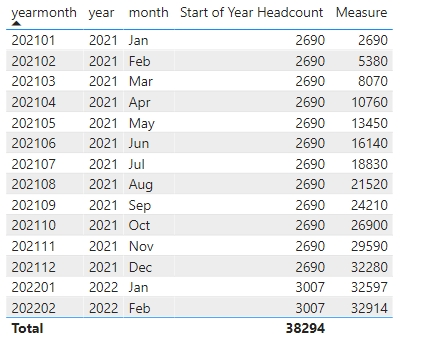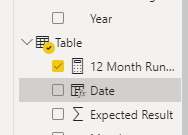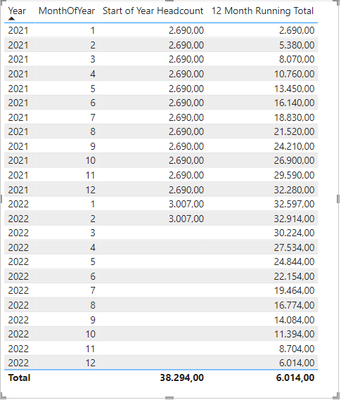cancel
Showing results for
Did you mean:Helper II

## Rolling 12 Month Headcount Sum as of Start of Year

Hi all,

I am stuck once again! My goal is to create a Rolling 12 Month Headcount Sum measure as of the start of the year. For example, headcount as of 1/1/2021 was 2,690. I want to apply this to every month in 2021 and then sum for those 12 months resulting in a total of 32,280. To complicate this a little bit more here is another example. Since it is February 2022, I would want to calculate a sum of the last 12 months knowing that the start of year headcount was 2,690 in 2021 and is now 3,007 in 2022. The expected measure would sum 2 months of 2022 at 3,007 headcount and 10 months of 2021 at 2,690 for a total of 32,914. I hope this makes sense as I have a difficult time trying to describe this.

Here is a table of my data:

 Year Month Start of Year Headcount Rolling 12 Month Headcount Sum Expected Result 2021 Jan 2,690 2021 Feb 2,690 2021 Mar 2,690 2021 Apr 2,690 2021 May 2,690 2021 Jun 2,690 2021 Jul 2,690 2021 Aug 2,690 2021 Sept 2,690 2021 Oct 2,690 2021 Nov 2,690 2021 Dec 2,690 2,690 32,280 2022 Jan 3,007 3,007 32,597 2022 Feb 3,007 3,007 32,914

Here is the DAX for Start of Year Headcount and Rolling 12 Month Headcount Sum:

``Start of Year Headcount = CALCULATE ( COUNTROWS ( Headcount ), STARTOFYEAR ( 'Date'[Date] ) )``
``````Rolling 12 Month Headcount Sum = CALCULATE (
DATESBETWEEN (
'Date'[Date],
NEXTDAY ( SAMEPERIODLASTYEAR ( LASTDATE ( 'Date'[Date] ) ) ),
LASTDATE ( 'Date'[Date] )
)
)``````

15 REPLIES 15Helper II

I should clarify. My Date table is at the day level. In the table, above I am using a date hierarchy. The trick here is that Headcount table is at the month end and start of year level. This means there are 13 dates in a completed year. I want to return the start of year value for each month in a year, sum this up and divide by 12 for the number of months in a year. I think the issue I am having is that I am filtering the Start of Year Headcount to the first day of the year. When I use other time intelligence functions it is considering only the first day of the year so it doesn't sum the start of year value for 12 months. This is pretty confusing so I apologize if this is not clear.Community Support

Hi @CatManKuhn ,

You could try edate() function.

Check this measure:

``Measure = SUMX(FILTER(ALLSELECTED('Table'),'Table'[date]>EDATE(SELECTEDVALUE('date'[date]),-12)&&'Table'[date]<=SELECTEDVALUE('date'[date])),'Table'[Start of Year Headcount])``Best Regards,

Jay

Community Support Team _ Jay
If this post helps, then please consider Accept it as the solution
to help the other members find it.Helper II

This works perfectly in your example, but I don't get any values when I enter this. It is entirely null. Any ideas why this may be?

``Measure Test = SUMX(FILTER(ALLSELECTED('Headcount'),'Headcount'[Date]>EDATE(SELECTEDVALUE('Date'[Date]),-12)&&'Headcount'[Date]<=SELECTEDVALUE('Date'[Date])),'Headcount'[Start of Year Headcount])``

Thank you!!Community Support

Hi @CatManKuhn ,

Not very certain. I created the demo based on the data you shared above. Is it possible that some conditions were ignored? You may try the below formula again. If it still doesn't work, please share the pbix.

``Measure Test = SUMX(FILTER(ALL('Headcount'),format('Headcount'[Date],"YYYYMM")>format(EDATE(SELECTEDVALUE('Date'[Date]),-12),"YYYYMM")&&format('Headcount'[Date],"YYYYMM")<=format(SELECTEDVALUE('Date'[Date]),"YYYYMM")),'Headcount'[Start of Year Headcount])``

Best Regards,

Jay

Community Support Team _ Jay
If this post helps, then please consider Accept it as the solution
to help the other members find it.Helper II

I will work to upload a pbix file. I will have to create a fake data set as this is sensitive data that I cannot share as it currently exists. What is the relationship betweent the Date and Headcount tables in your example? Is it joined on Date[Date] and Headcount[Date]?

Thanks!Super User

Hi,

Try creating this measure

Date = DATEVALUE("01-"&'Table'[Month]&"-"&'Table'[Year])

Then with this measure all function:

12 Month Running Total =
Var SelectedMaxDate = MAX ( Dates[Date] )
Var MinDate =
CALCULATE (
MIN ( Dates[Date] ),
FILTER (
ALL ( Dates ),
Dates[Date],
1,
YEAR
) >= SelectedMaxDate
)
)
Return
CALCULATE (
SUM ( 'Table'[Start of Year Headcount] ),
ALL ( Dates ),
Dates[Date] <= SelectedMaxDate,
Dates[Date] >= MinDate
)

I hope this solution is able to help you to solve your issue and if it does consider giving the post a thumbs up or accept it as a solution!Helper II

Thank you for the response. The Date measure results in an error. I tried the other measure, but it only returns the start of year headcount value.Super User

Sorry but i forgot to insert the measure:

12 Month Running Total =
Var SelectedMaxDate = MAX ( Dates[Date] )
Var MinDate =
CALCULATE (
MIN ( Dates[Date] ),
FILTER (
ALL ( Dates ),
Dates[Date],
1,
YEAR
) >= SelectedMaxDate
)
)
Return
CALCULATE (
SUM ( 'Table'[Start of Year Headcount] ),
ALL ( Dates ),
Dates[Date] <= SelectedMaxDate,
Dates[Date] >= MinDate
)

Regarding the date maybe it depends on your local formatfor date? Be sure that Date is a calculated columnHelper II

Where are you creating the Date column? On the date table, headcount table (where Start of Year headcount exists), or a new table? I am confused by the "link it to your date table" statement.

Thanks!Super User

Hi,

this is the model.My values and tableMy column date in tableDate = DATEVALUE("01-"&'Table'[Month]&"-"&'Table'[Year])
and MonthInt in DatesThat's all.

I try to study if it is possible to share the PbixSuper User

Hi,

this is the result i obtained.To do this i create a query:

let
Source = Table.FromRows(Json.Document(Binary.Decompress(Binary.FromText("fdFBC8IgGAbgv/Lh2YNz1doxqIjBCrZTjB1ssxWYjqVR/z63sfJQgvqCzwuKFgU6ctYhjFIl9cVmrlmnQZ2h34cdZ3WljNRWMiXEVTYQUBjKX4Tc3Gxh82x5pXkNGb8boVGJC0QJDSwlTNqVLmJiox+ObfmpNzwiDNPhdLjeX161Xk7Zy8eJkX4W3rNN4+Oct9rnh8rLe/Xw8ZpXDk8ZUkyXZOrRz9OHmJDITYrnceT2xm/40YuDGSrLNw==", BinaryEncoding.Base64), Compression.Deflate)), let _t = ((type nullable text) meta [Serialized.Text = true]) in type table [Year = _t, #"(blank)" = _t, #"(blank).1" = _t, #"(blank).2" = _t, #"(blank).3" = _t]),
#"Changed Type" = Table.TransformColumnTypes(#"Replaced Value",{{"Year", Int64.Type}, {"Month", type text}, {"Start of Year Headcount", type number}, {"Rolling 12 Month Headcount Sum", type number}, {"Expected Result", type number}})
in
#"Changed Type"

Then i used my usual query to create the date table and load to the model.

This is the relationship:Date = DATEVALUE("01-"&'Table'[Month]&"-"&'Table'[Year])

is a column in Table and "12 Month Running Total" is a mesure:Super User

Hi,

Try something like this:

`CALCULATE ( [Start of year headcount], DATESBETWEEN ( 'Calendar'[Date], DATEADD ( LASTDATE ( DATEADD ( 'Calendar'[Date], -12, MONTH ) ), +1, DAY ), LASTDATE ( 'Calendar'[Date] ) )  )`

I hope this post helps to solve your issue and if it does consider accepting it as a solution and giving the post a thumbs up!

Proud to be a Super User!Helper II

Thank you for the response. I tried your suggestion, but I get the same values as my Rolling 12 Month Headcount Sum measure.Super User

@CatManKuhn

What kind of relationship do you have between your fact table and calendar table?

Proud to be a Super User!Helper II

I have a many to one relationship between Headcount and Date.